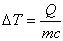Heat Q = mass m times specific heat c times change in temperature delta T

This equation is more easily understood if solved for delta T:When heat flows into an object or a sample of a substance, the temperature of that object or sample increases (as long as the object or sample is not at its melting point or boiling point). The amount by which the temperature increases depends on the amount of heat that flows into the object, the mass of the object, and a constant characterizing the material of which the object or sample is made. The constant is known as the specific heat of the substance.